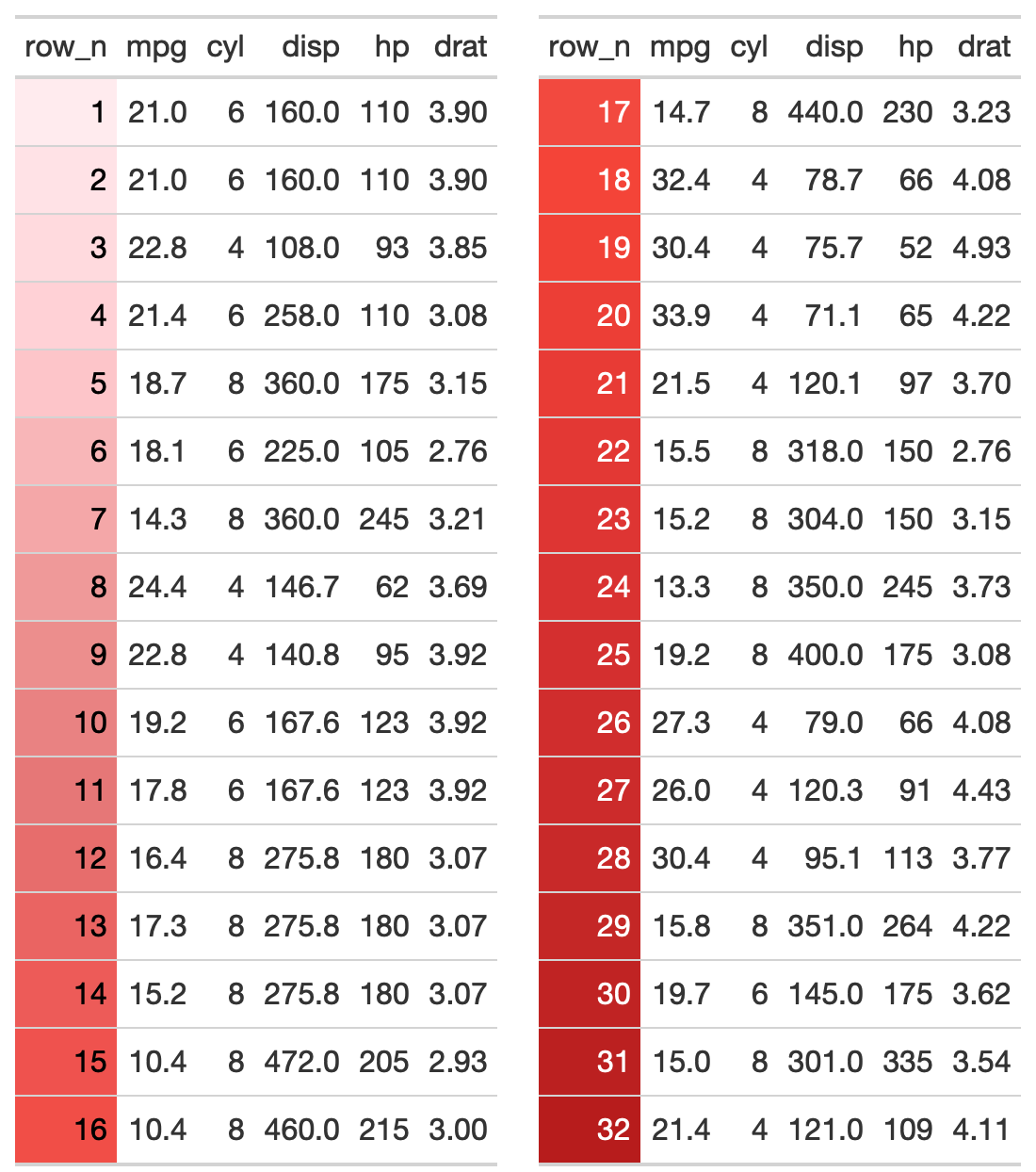This function takes a list() of two gt-tables and returns them as a two-column layout. The expectation is that the user either supplies two tables like list(table1, table2), or passes the output of gt_double_table() into this function. The user should indicate whether they want to return the HTML to R's viewer with output = "viewer" to "view" the final output, or to save to disk as a .png via output = "save". Note that this is a relatively complex wrapper around htmltools::div() + webshot2::webshot(). Additional arguments can be passed to webshot2::webshot() if the automatic output is not satisfactory. In most situations, modifying the vwidth argument is sufficient to get the desired output, but all arguments to webshot2::webshot() are available by their original name via the passed ....

## Usage

gt_two_column_layout(
tables = NULL,
output = "viewer",
filename = NULL,
path = NULL,
vwidth = 992,
vheight = 600,
...,
zoom = 2,
expand = 5
)

## Arguments

tables

A list() of two tables, typically supplied by gt_double_table()

output

A character string indicating the desired output, either "save" to save it to disk via webshot, "viewer" to return it to the RStudio Viewer, or "html" to return the raw HTML.

filename

The filename of the table, must contain .png and only used if output = "save"

path

An optional path of where to save the printed .png, used in conjunction with filename.

vwidth

Viewport width. This is the width of the browser "window" when passed to webshot2::webshot().

vheight

Viewport height This is the height of the browser "window" when passed to webshot2::webshot().

...

Additional arguments passed to webshot2::webshot(), only to be used if output = "save", saving the two-column layout tables to disk as a .png.

zoom

Argument to webshot2::webshot(). A number specifying the zoom factor. A zoom factor of 2 will result in twice as many pixels vertically and horizontally. Note that using 2 is not exactly the same as taking a screenshot on a HiDPI (Retina) device: it is like increasing the zoom to 200 doubling the height and width of the browser window. This differs from using a HiDPI device because some web pages load different, higher-resolution images when they know they will be displayed on a HiDPI device (but using zoom will not report that there is a HiDPI device).

expand

Argument to webshot2::webshot(). A numeric vector specifying how many pixels to expand the clipping rectangle by. If one number, the rectangle will be expanded by that many pixels on all sides. If four numbers, they specify the top, right, bottom, and left, in that order. When taking screenshots of multiple URLs, this parameter can also be a list with same length as url with each element of the list containing a single number or four numbers to use for the corresponding URL.

## Value

Saves a .png to disk if output = "save", returns HTML to the viewer via htmltools::browsable() when output = "viewer", or returns raw HTML if output = "html".

## Examples

Add row numbers and drop some columns

library(gt)
my_cars <- mtcars %>%
dplyr::mutate(row_n = dplyr::row_number(), .before = mpg) %>%
dplyr::select(row_n, mpg:drat)

Create two tables, just split half/half

tab1 <- my_cars %>%
dplyr::slice(1:16) %>%
gt() %>%
gtExtras::gt_color_rows(columns = row_n, domain = 1:32)

tab2 <- my_cars %>%
dplyr::slice(17:32) %>%
gt() %>%
gtExtras::gt_color_rows(columns = row_n, domain = 1:32)

Put the tables in a list and then pass list to the gt_two_column_layout function.

listed_tables <- list(tab1, tab2)

gt_two_column_layout(listed_tables)

A better option - write a small function, use gt_double_table() to generate the tables and then pass it to gt_double_table()

my_gt_fn <- function(x) {
gt(x) %>%
gtExtras::gt_color_rows(columns = row_n, domain = 1:32)
}

my_tables <- gt_double_table(my_cars, my_gt_fn, nrows = nrow(my_cars) / 2)

This will return it to the viewer

gt_two_column_layout(my_tables)

If you wanted to save it out instead, could use the code below

gt_two_column_layout(my_tables, output = "save",
filename = "basic-two-col.png",
vwidth = 550, vheight = 620)

## FiguresOther Utilities: add_text_img(), fa_icon_repeat(), fmt_pad_num(), fmt_pct_extra(), fmt_symbol_first(), generate_df(), gt_add_divider(), gt_badge(), gt_double_table(), gt_duplicate_column(), gt_fa_column(), gt_fa_rank_change(), gt_fa_rating(), gt_fa_repeats(), gt_highlight_cols(), gt_highlight_rows(), gt_img_border(), gt_img_circle(), gt_img_multi_rows(), gt_img_rows(), gt_index(), gt_merge_stack_color(), gt_merge_stack(), gtsave_extra(), img_header(), pad_fn(), tab_style_by_grp()